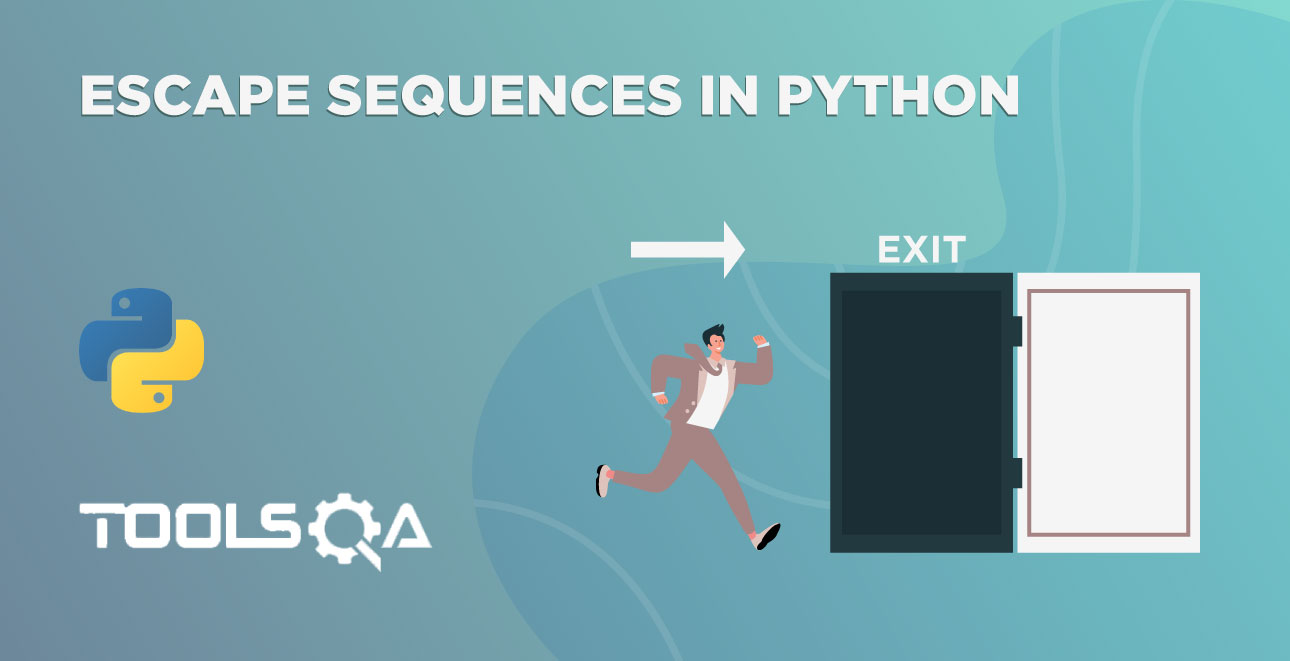Congratulations! You have completed the first module of the course. In addition to this, you know how to run code using different editors. We executed print function in Python but didn't discuss it's functionality. In this tutorial, we are going to see the print function in detail. We will discuss the topics below.

• What is the Python Print Function?
• Empty print() statement
• Single Argument
• Keyword Argument - End
• Multiple Arguments
• Keyword Argument - Sep

## What is Python Print Function?

The word print is a Function in Python.

### What is a function?

A function is a block of code that can perform a specific action. In addition to this, these block of code doesn't execute until you call or invoke the function. Majorly functions are created with the hope that we will use the same code multiple times. For example, you have to find the sum of two numbers for ten different combinations. So, it's better to define a function instead of writing the same code for all these combinations.

There are two types of Functions in Python

• Built-in functions - The functions that are pre-defined in Python are known as Built-in functions.
• User-defined functions - The functions that are created by the programmers or developers in Python are called User-defined functions.

We will discuss functions in detail in later articles. For now, you must have figured out that print is a Built-in function!

A function can affect something or gives a result. Let's take an example to understand the effect and result.

• Let's say we have a function called create_folder in an operating system. In other words, its functionality is to create folders. Moreover, the effect caused by create_folder is generating a new folder. Some more examples are sending a message, opening an app, closing a window, etc.
• In the same vein, let's take an example of the Sum Function. It gives a result back to us by adding the numbers.

Here comes a million-dollar question, how does the sum function get those numbers for the addition? Some of you think we will define them in the function. Yeah, that's also an answer. But, most probably, we will give the numbers to the function while invoking it. That is to say, the numbers that we are passing to the function are known as arguments. Therefore, the function will take that argument and give the result based on what arguments pass.

E.g. SumMyNumbers(2,3) , SumMyNumbers(3,4) etc.

Note - SumMyNumbers is a user-defined function that will return a result. Additionally, it will be the sum of arguments that you have passed to it.

For a quick note, arguments are the data that a function needs to perform some operations. In the SumMyNumbers example, it needs numbers as data. Some other function needs a String, e.g., "Hello World". It depends on case to case. We will talk about the functions and arguments in later tutorials.

Now that we got some idea on function, let's get back to print function.

The function print() takes zero or more arguments and displays them on the screen. Moreover, it takes any data. The print() is sending the data to an output device, i.e., console. Sending the data to the console is the effect caused by print() function. The print() function does not evaluate anything.

### Empty print() Statement

What will we get when we call the Python print function without any arguments? Do we get any errors? No ! We won't get any error with an empty print() statement. It simply prints a new line.

Let's see it practically. Open the IDE and run the following code:

``````# empty print statement
print()
``````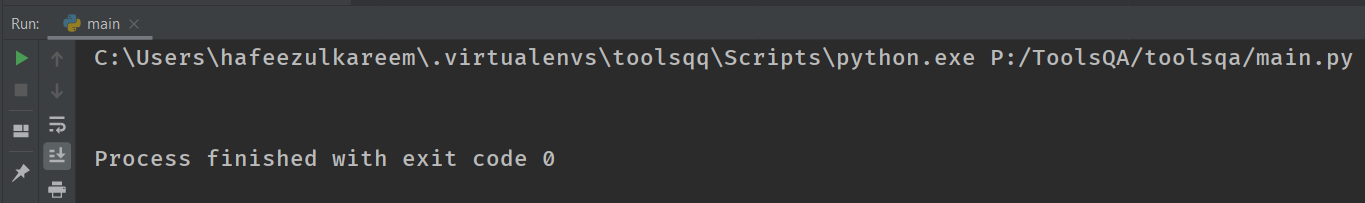Did you see any results? No? Look closely. Did you realize that there is a new line? We will demonstrate the presence of a new line in the next section with three print statements in the latter part of the article.

### Single Argument

We had already seen a single argument when we used print function to print "Hello World". It displays or outputs the data that you pass to the print() function. Moreover, the syntax is print(data). If we pass a single argument with quotes, it prints that value, as we saw in the hello_world program.

Let's see another example.

``````# argument with quotes
print("ToolsQA")
``````

Note: You can also use the single-quotes in place of double-quotes. In other words, both work the same in Python.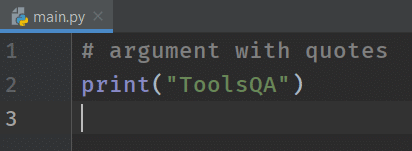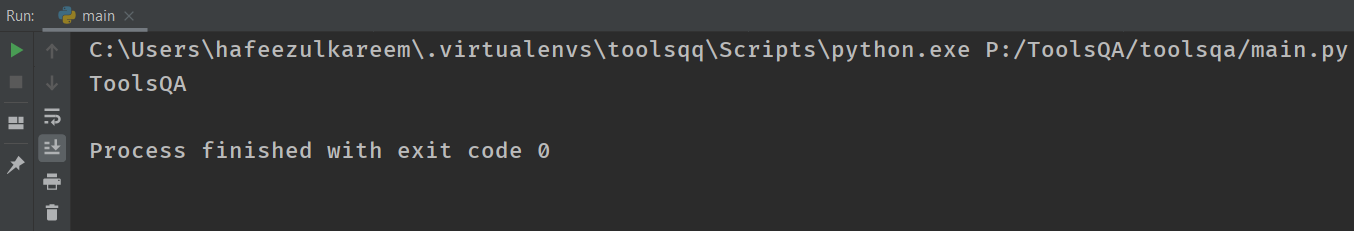We got ToolsQA as output. I think by now you could already figure this out.

What happens if we pass the "ToolsQA" without quotes? Try it yourself.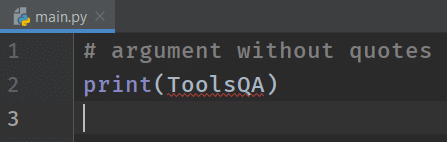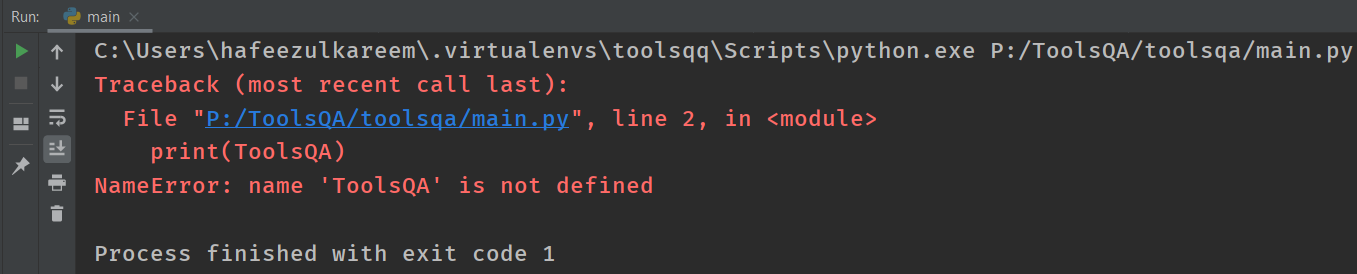We got an error. The error is "name 'ToolsQA' is not defined". What's that error, and how can we resolve it? It is related to the concept of literals and variables. We will study it in detail in subsequent articles, however, let's quickly touch base it here.

Literal is data that can be identified by itself. In other words, you can think of it as a value. For example, if you take a number 759, you can quickly recognize it by merely seeing it. Now consider x, what do you recognize from that? Here x is a variable, which stores the values. Moreover, the value can be anything. So, x here can be 10 or 20 or any other value.

"ToolsQA" is a literal whereas ToolsQA is a variable. That is to say, when you enclose a data in quotes, it becomes a String (more on that in later chapters !)

We have discussed that the empty print() function prints a new line. To demonstrate it, write three print statements as you wish. But leave the second print statement empty.

Take the following code as a reference:

``````# demonstrating new line with empty print statement
print("ToolsQA")
print()
print("Python PCEP Course")
``````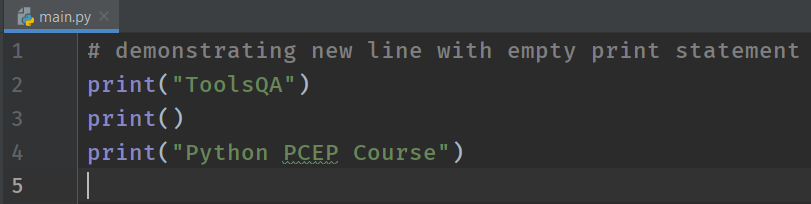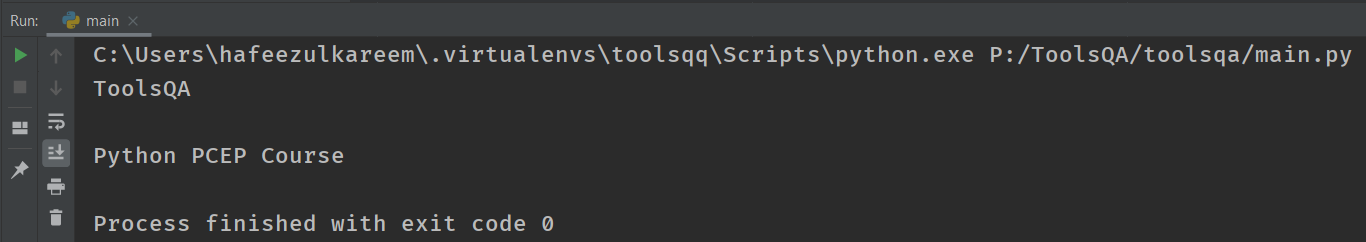You can see a line in between the "ToolsQA" and "Python PCEP Course". As we mentioned, the empty print statements give us a new line.

What happens when we pass multiple lines as an argument to the print function. Let's see it.

``````# multiple lines in a print statement
print("ToolsQA
Python PCEP Course")
``````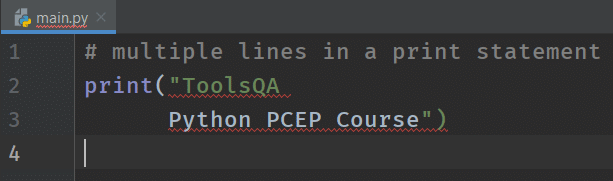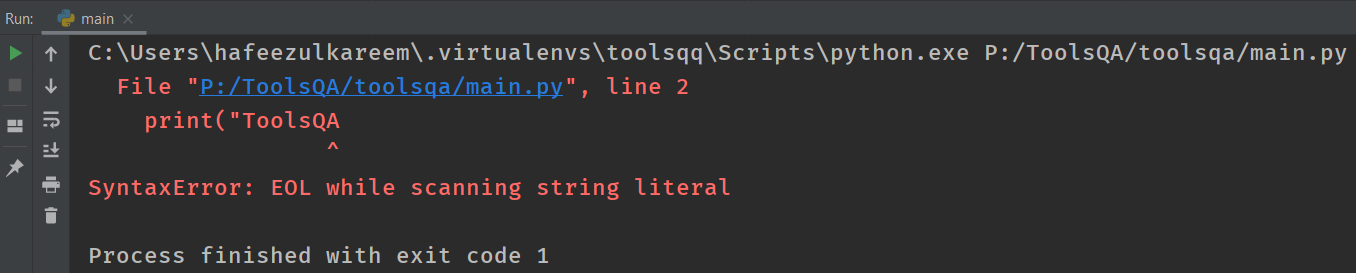We got an error. Can we do anything to resolve it? Yeah! Of course, we can. Consequently, add quotes at the end of the first-line text and start of the second-line text. You can see the code in the block below.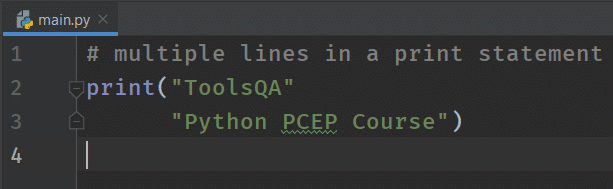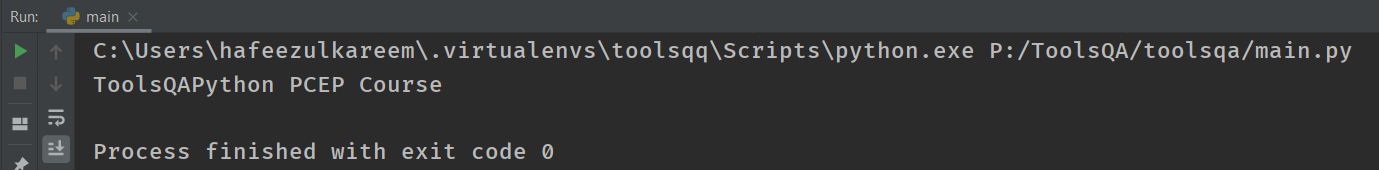### Keyword Argument - END in Print Function

Before we move further, let's understand one quick concept about End Argument that can pass to print function. We will study it in detail later as part of the keyword arguments, but let's quickly touch base on it. If we get back to our earlier example:

``````print("ToolsQA")
print("Python PCEP Course")
``````

you will see that the output prints in 2 lines. But what if we have to get it printed in the same line, even though we use two print functions? As a result, we pass the End Argument, which ensures the output receives no newline. Now, try this on your own

``````print("ToolsQA", end=" ")
print("Python PCEP Course")
``````

Did you get it in the same line? You surely did!

### Multiple Arguments in Python Print Function

What if we pass more than one argument to the print function? Consequently, it prints all the values with a space between them. We have to pass the arguments with a comma in between them, which acts as a separator. For example, if you have given the numbers 2, 5 to the print function, then the output you will get is 2 5. Let's see it using an example.

``````# passing multiple arguments
print(2, 5)
``````

Run the above program in PyCharm: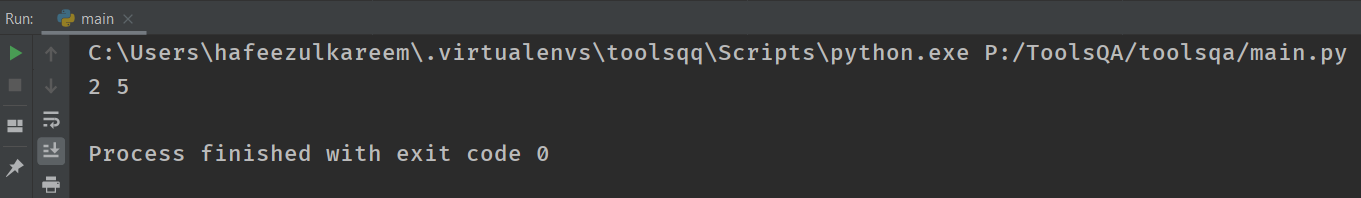We can pass as many arguments as we want and any argument.

Let's see what happens when we pass multiple texts to the print function.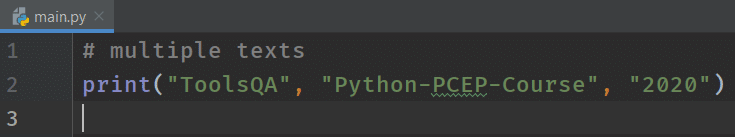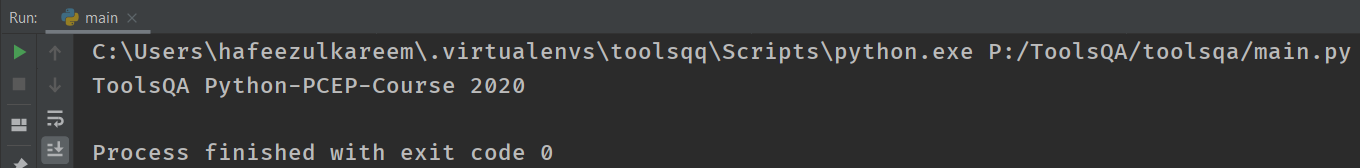We got the output in the same line as the previous program. Now try below on your own -

print ("Tools" "QA") - See what happens when we don't have "" in-between? You got it right. There is no space in the output now. As a result, you will get ToolsQA.

### Keyword Argument - SEP in Print Function

A sep argument alters the behavior of the print function. Additionally, here, instead of space, you get what passes in sep argument. Try this code

print (2,5,6) -  You will obviously get 2 5 6 as output*.

Now Try

print(2,5,6, sep="") - You will get 256 as output*.

## Key Takeaways

• The print() function sends the data to the output console.
• Moreover, we get a space between the data as default for multiple arguments. You can change it using the optional argument sep.
• print() function prints a newline at the end of the output as default. Additionally, you can change it using the optional argument end.
• An empty print() function prints a newline.

## Practice Yourself

``````print("ToolsQA")
``````
``````print("ToolsQA", "2019")
``````
``````print("ToolsQA", "2019", sep="@")
``````
``````print("ToolsQA")
``````
``````print(2019)
``````
``````print("ToolsQA", end='')
``````
``````print(2019)
``````
``````print("Welcome to ToolsQA", "2020", sep="@", end="!")
``````

## What Next?

To conclude, practice the print statement to get familiar with its behavior. And remember the print function is what we are going to use a lot in this course. In addition to this, try to print a backslash in your IDE. Do you get the output? No! I know. It's not the way to print a backslash. Curious to know? We will see escape sequences in Python in the next tutorial.ppy Coding :)